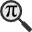## General

Display information for equation id:math.249581.2 on revision:249581

* Page found: Pendulum (mathematics) (eq math.249581.2)

(force rerendering)

Cannot find the equation data in the database. Fetching from revision text.

Occurrences on the following pages:

Hash: ee5e5c003694e7cd5ae404923c665edb

TeX (original user input):

\ell


TeX (checked):

\ell


### LaTeXML (experimental; uses MathML) rendering

MathML (499 B / 259 B) :${\displaystyle\ell}$
<math xmlns="http://www.w3.org/1998/Math/MathML" id="p1.1.m1.1" class="ltx_Math" alttext="{\displaystyle\ell}" display="inline">
<semantics id="p1.1.m1.1a">
<mi mathvariant="normal" id="p1.1.m1.1.1" xref="p1.1.m1.1.1.cmml">ℓ</mi>
<annotation-xml encoding="MathML-Content" id="p1.1.m1.1b">
<ci id="p1.1.m1.1.1.cmml" xref="p1.1.m1.1.1">ℓ</ci>
</annotation-xml>
<annotation encoding="application/x-tex" id="p1.1.m1.1c">{\displaystyle\ell}</annotation>
</semantics>
[/itex]


SVG (1.162 KB / 687 B) :

### MathML with SVG or PNG fallback (recommended for modern browsers and accessibility tools) rendering

SVG image empty. Force Re-Rendering

SVG (0 B / 8 B) :

PNG (0 B / 8 B) :

$\ell$## Translations to Computer Algebra Systems

### Translation to Maple

In Maple: ell

\ell: was translated to: ell

### Translation to Mathematica

In Mathematica: \[ScriptL]

\ell: was translated to: \[ScriptL]

## Similar pages

Calculated based on the variables occurring on the entire Pendulum (mathematics) page

## Identifiers

• $\ell$### MathML observations

0results

0results

no statistics present please run the maintenance script ExtractFeatures.php

0 results

0 results